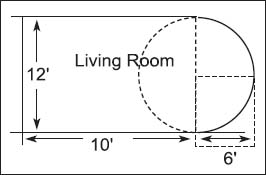NextPrevious

# How can a person calculate the amount of carpeting needed to redecorate a room in a house?

Watching remodeling programs on television, visiting someone else’s house, or even getting cabin fever in the winter often triggers the need to redecorate from floor to ceiling. But just how much new carpeting does a house need? The main way to discover how to cover up the floors is by using simple geometry, the branch of mathematics dealing with area, distance, volume, and the properties of shapes and lines. (For more about geometry, see “Geometry and Trigonometry.”)

For example, if a person needs to buy new carpeting for a bedroom, just use the formula A = L × W, or the area equals the length times the width. For a bedroom that measures 10 feet by 12 feet, the area would equal 120 square feet. Thus, the room would need 120 square feet of carpeting.

If a living room has a circular alcove, there is another measurement one can use: A = pi (π) × r2, or the area (A) equals pi times the radius squared. If the room measures 12 feet in width at the long end of the alcove (or the diameter of the alcove), the radius of the alcove is 6 feet. Thus, A = 3.14159 × (6)2, or 113 square feet (rounded to the nearest square foot), would equal the area of an entire circle. Because an alcove is half of a circle, divide the 113 square feet in half, or about 56.5 square feet. Add this area to the rest of the room. For instance, if the rest of the room measures 10 by 12 feet, multiply 10 × 12 = 120, then add the alcove area of 56.6 to get the needed amount of carpeting: 176.5 square feet.Measuring a room for a circular rug involves some basic knowledge of mathematics.

Close

This is a web preview of the "The Handy Math Answer Book" app. Many features only work on your mobile device. If you like what you see, we hope you will consider buying. Get the App